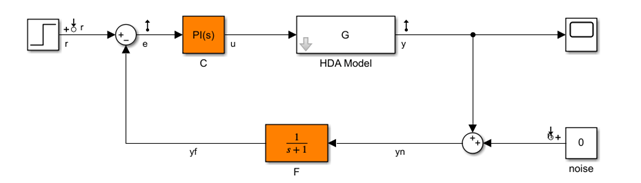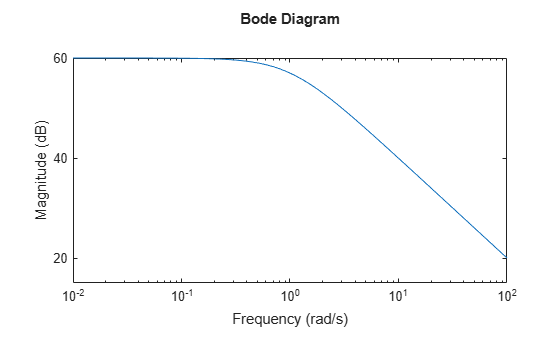# Extract Tunable Control System from Simulink Model

This example shows how to create a tunable model for tuning with `hinfstruct`, starting with a Simulink® model of your control system. To do so:

1. Create an `slTuner` interface to the model, specifying the model elements that are tunable.

2. Extract a linearized, tunable model from the `slTuner` interface.

3. Define weighting functions that capture your design requirements, and incorporate them into the tunable model.

### Create `slTuner` Interface

Open the model and create the `slTuner` (Simulink Control Design) interface. The interface parameterizes the tunable blocks you specify, and allows you to extract linearized open-loop and closed-loop responses from the model. For this example, use the `rct_diskdrive` model.

`open_system('rct_diskdrive')`The model includes a plant model, a PI controller, and a low-pass filter in the feedback path. The model is also preconfigured with several analysis points: outputs at the error signal `e` and the plant output `y`, and additive inputs for the reference command and measurement noise. To create the `slTuner` interface, specify the tunable blocks.

`ST0 = slTuner('rct_diskdrive',{'C','F'});`

The `slTuner` interface automatically parametrizes these blocks using a PI controller for `C` and a transfer function with two free parameters for `F`. Because F is a low-pass filter, specify a custom parameterization to further constrain its coefficients.

```a = realp('a',1); setBlockParam(ST0,'F',tf(a,[1 a]));```

### Extract Tunable Closed-Loop Model

Use `getIOTransfer` to obtain a tunable model of the closed-loop transfer function from the reference and noise inputs `{'r','n'}` to the measurement and error outputs `{'y','e'}`.

```T0 = getIOTransfer(ST0,{'r','n'},{'y','e'}); T0```
```Generalized continuous-time state-space model with 2 outputs, 2 inputs, 11 states, and the following blocks: C: Tunable PID controller, 1 occurrences. a: Scalar parameter, 2 occurrences. Type "ss(T0)" to see the current value and "T0.Blocks" to interact with the blocks. ```

This command returns a generalized LTI (`genss`) model with tunable blocks `C` and `F`. (For more information about tunable models, see Models with Tunable Coefficients.)

### Append Weighting Functions

For `hinfstruct`, encode the desired response with the weighting functions that express a target loop shape `LS`.Appending the transfer function `LS` at the error output and `1/LS` at the noise input. Let T(s) denote the closed-loop transfer function from the inputs `{'r','nw'}` to the outputs `{'y','ew'}`. Then, constraining the H-infinity norm of T to less than 1 $\left({‖\mathit{T}\left(\mathit{s}\right)‖}_{\infty \text{\hspace{0.17em}}}<1\right)$ approximately enforces the target loop shape. (See Formulating Design Requirements as H-Infinity Constraints.)

For this example, use the target loop shape given by:

`$\mathrm{LS}=\frac{1+0.001\left(\mathit{s}/{\omega }_{\mathit{c}}\right)}{0.001+\left(\mathit{s}/{\omega }_{\mathit{c}}\right)},{\text{\hspace{0.17em}}\omega }_{\mathit{c}}=1000$`

This value of LS corresponds to the following open-loop response shape.

```wc = 1000; s = tf('s'); LS = (1+0.001*s/wc)/(0.001+s/wc); bodemag(LS)```Append LS to the transfer function extracted from the `slTuner` interface to create the complete tunable weighted control system model.

`T0 = blkdiag(1,LS) * T0 * blkdiag(1,1/LS);`

You can now use `hinfstruct` to tune the `a` and the parameters in `C`. See Tune and Validate Controller Parameters.